# Citigroup

## Analyst Listing

The following analysts provide coverage for the subject firm as of May 2016:

 Broker Analyst Analyst Email Keefe Bruyette & Woods Brian Kleinhanzl bkleinhanzl@kbw.com Oppenheimer Chris Kotowski chris.kotowski@opco.com Atlantic Equities Christopher Wheeler c.wheeler@atlantic-equities.com Drexel Hamilton David Hilder dhilder@drexelhamilton.com Guggenheim Securities Eric Wasserstrom eric.wasserstrom@guggenheimpartners.com RBC Capital Markets Gerard Cassidy gerard.cassidy@rbccm.com Evercore ISI Glenn Schorr glenn.schorr@evercoreisi.com BMO Capital Markets James Fotheringham james.fotheringham@bmo.com Buckingham Research James Mitchell jmitchell@buckresearch.com Sandler O’Neill & Partners Jeffery J. Harte jharte@sandleroneill.com Bernstein Research John E. McDonald john.mcdonald@bernstein.com Jefferies Kenneth Usdin kusdin@jefferies.com Wells Fargo Securities Matthew H. Burnell matt.burnell@wellsfargo.com Deutsche Bank Research Matthew O’Connor matthew.o-connor@db.com Societe Generale Murali Gopal murali.gopal@sgcib.com Nomura Research Steven Chubak Steven.Chubak@nomura.com Credit Suisse Susan Roth Katzke susan.katzke@credit-suisse.co

## Primary Input Data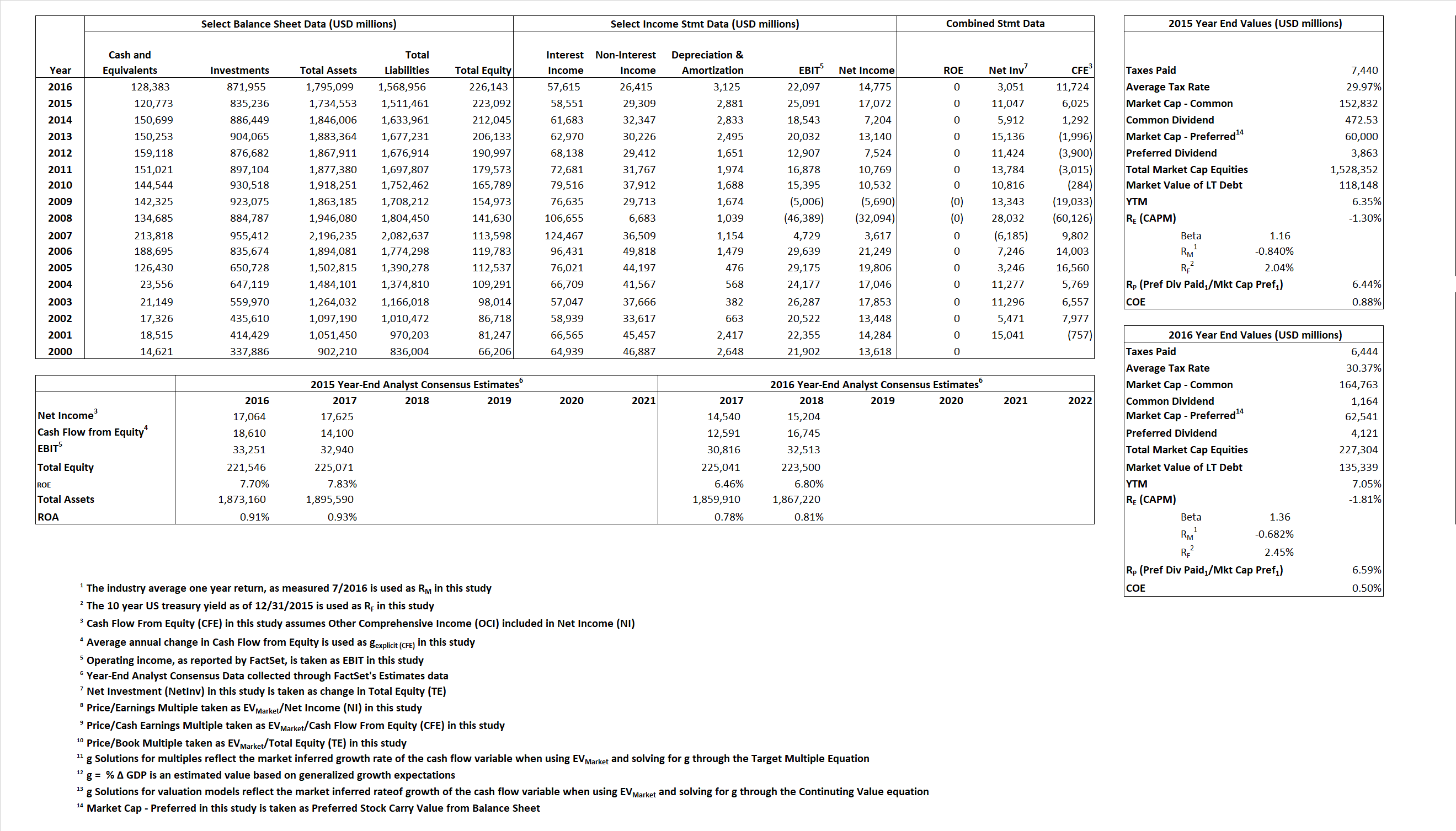## Derived Input Data

### Equational Form

Net Income NI$NI = \, EBIT\, -\, Interest\, - \,Taxes$
Cash Flow From Equity CFE$FCF\,=\,NOPLAT - \Delta \,TE\, + \,Other\,\, Comprehensive\,\, Income$
Total Equity TE$TE\,=\, Paid \,\,in\, Equity\,\,Capital\,+\, Accumulated\,\,Retained\,\,Earnings$
Return on Equity ROE$ROE \,=\,\frac { NI }{TE }$
Net Investment NetInv$NetInv\,=\,\Delta\,\,TE\,=\,{TE}_{1}-{TE}_{0}$
Investment Rate IR$IR\,=\,\frac {NetInv}{NI}$
Cost of Equity COE$COE\,=\,{R}_{F}\,x \,({R}_{M}-{R}_{F})\, x\, Beta$
Enterprise value EVMarket$EV\,=\,Market\,\,Cap\,\,Equity\,+\,Market\,\, Value\,\,Long\,\,Term\,\,Debt\,-\,Cash$
EVBook
EV/EBIT Multiple$\frac{EV_{Market}}{EBIT}$$EV/EBIT\,=\,\frac { EV}{ EBIT}$
Long-Run Growth g = %$\Delta$ GDP   Long-run growth rates of the income variable (g = IR x ROIC and g = %$\Delta$ GDP) are used in the Continuing Value portion of the valuation models.
g = IR x ROIC

## Valuation Model Outcomes

The outcomes presented in this study are the result of original input data, derived data, and synthesized inputs and, depending on the equational form of any particular valuation model, may result in irrelevant or implausible results.  For example, in the event WACC < g, the value of this term, often found in the denominator of an equation’s continuation value term, will be expressly negative and may result in a negative overall valuation for the firm.  In the event of a WACC < g relation, the model form as applied to the subject firm offers an irrelevant outcome.

### Equational form

Key Value Driver (NI) KVD (NI)${ Value }_{ DCF/KVD }=\sum { \frac { NI_{ t } }{ { \left( 1+COE \right) }^{ t } } +\frac { \frac { { NI }_{ 1 }\left( 1-\frac { g }{ ROE } \right) }{ COE-g } }{ { \left( 1+COE \right) }^{ t } } }$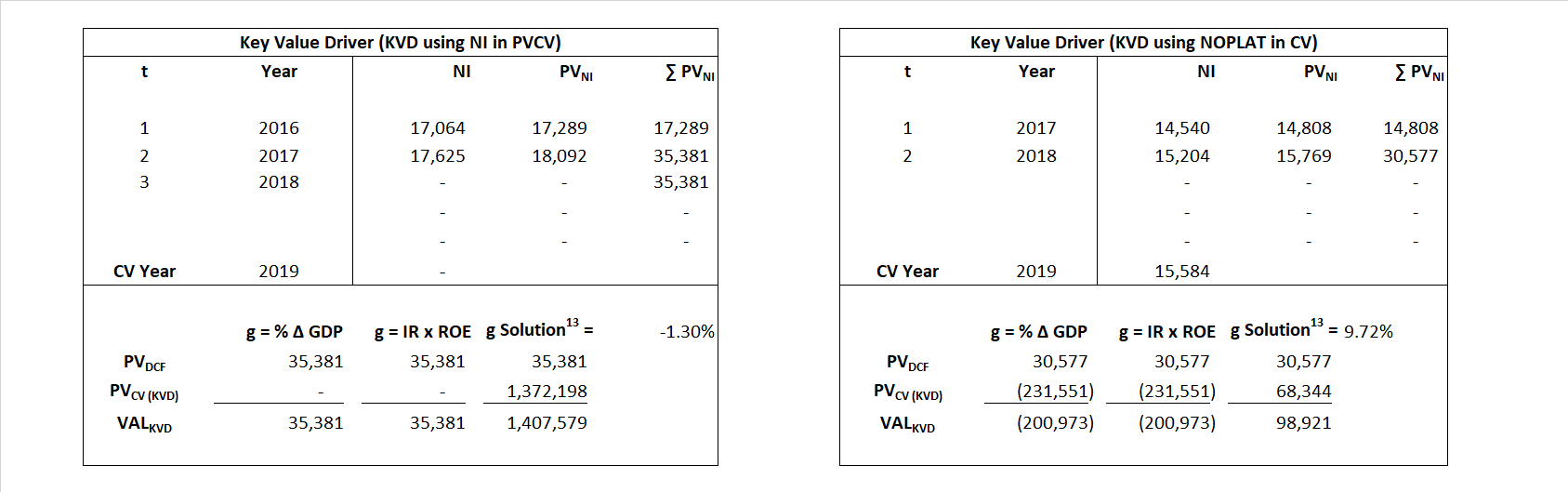Key Value Driver (CFE) KVD (CFE)${ Value }_{ DCF/KVD }=\sum { \frac { CFE_{ t } }{ { \left( 1+COE\right) }^{ t } } +\frac { \frac { {NI}_{ 1 }\left( 1-\frac { g }{ ROE } \right) }{COE-g } }{ { \left( 1+COE \right) }^{ t } } }$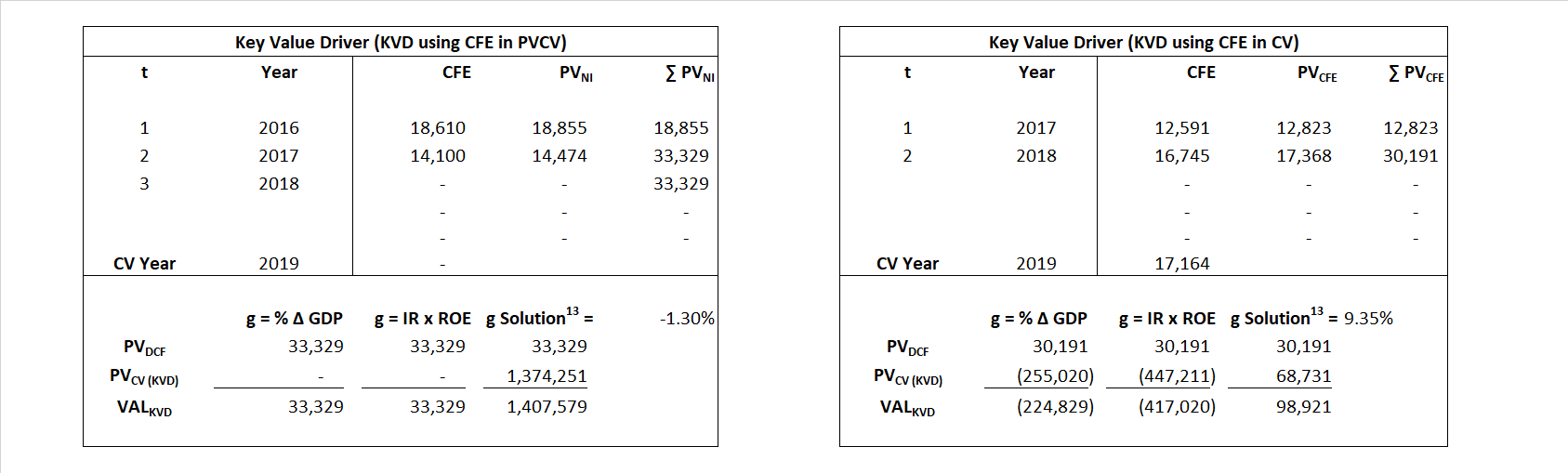Cash Flow From Equity CFE${ Value }_{ DCF/CFE }=\sum { \frac {CFE_{ t } }{ { \left( 1+COE \right) }^{ t } } +\frac { \frac { {CFE }_{ 1 }}{ COE-g } }{ { \left( 1+COE \right) }^{ t } } }$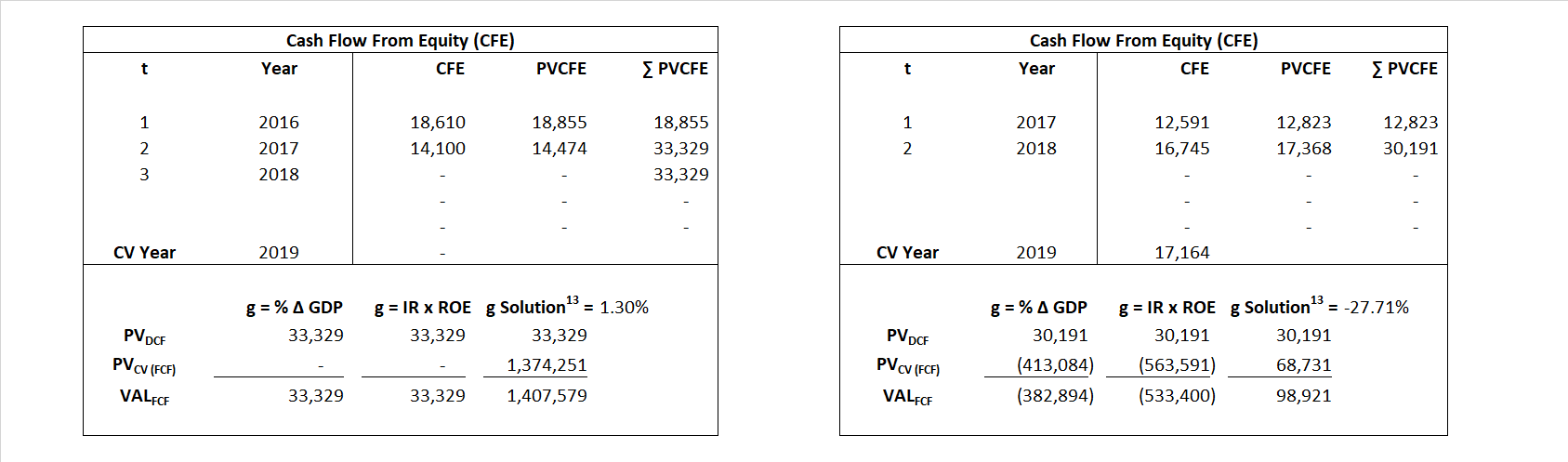Economic Profit ECON π${ Value }_{ { ECON\pi } }= {TE}_{ 0 }+\sum { \frac { {TE}_{ t-1 }({ROE}_{t}-{COE}_{t}) }{ { \left( 1+COE \right) }^{ t } }+ \frac {\frac {{TE}_{0}\ x\ ({ROE}_{1}\ -\ {COE}_{1}) }{COE-g } }{ { \left( 1+COE\right) }^{ t } } }$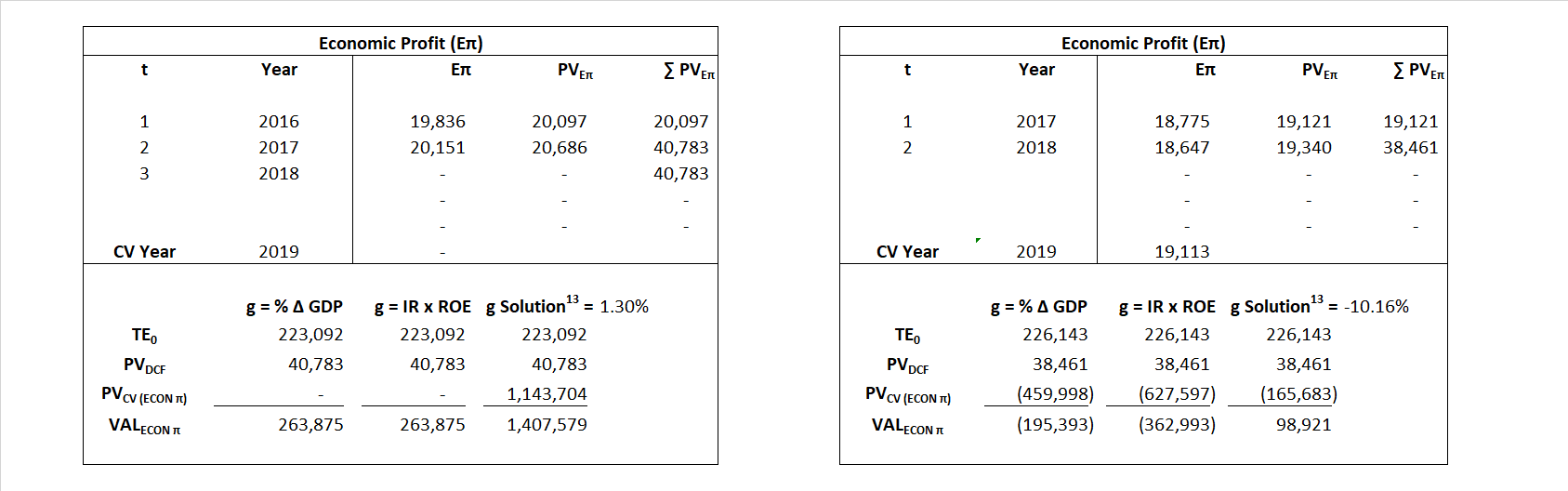Forward Market Multiple FMM${ Value }_{ DCF/FMM}=\sum { \frac { CFE_{ t } }{ { \left( 1+WACC \right) }^{ t } } +\frac { { EBIT }_{ 1 }\,{x}\,{FMM}}{ { \left( 1+WACC \right) }^{ t } } }{\,\,\,; \,\,FMM\,=\,\frac{{EV}_{t=0}}{{EBIT}_{t=0}}}$